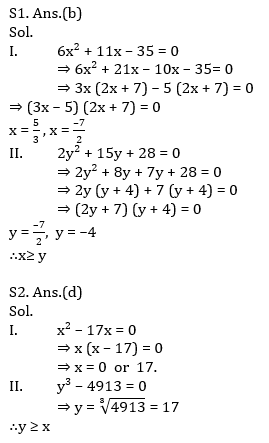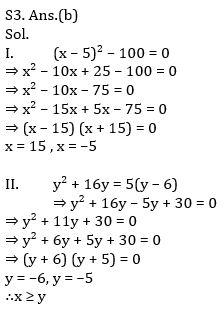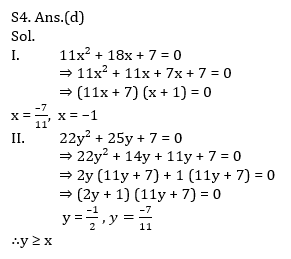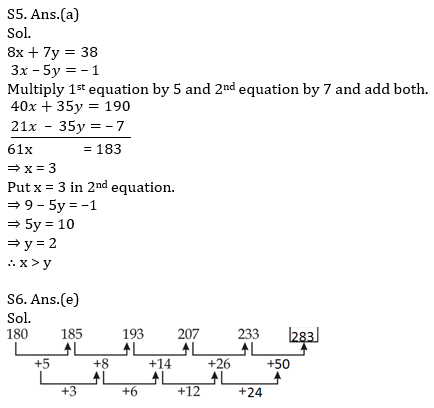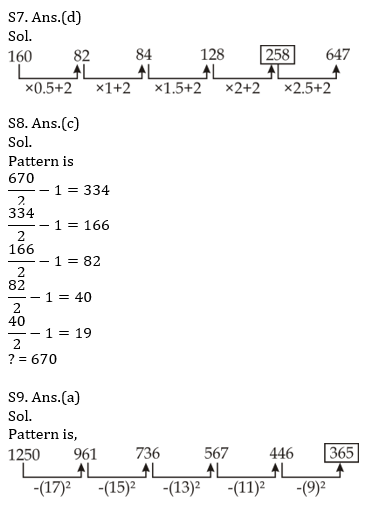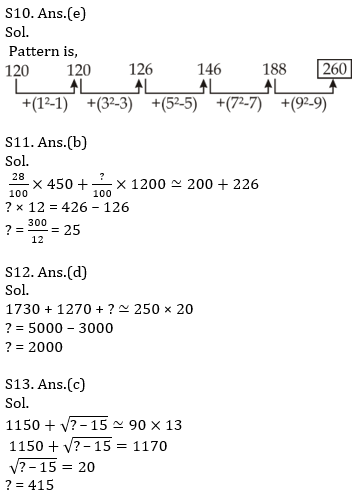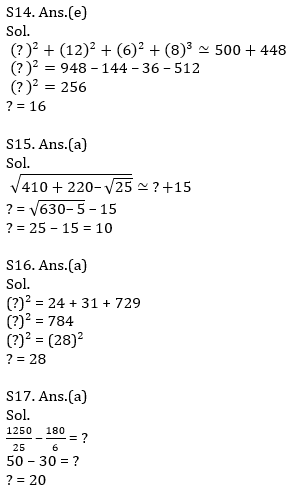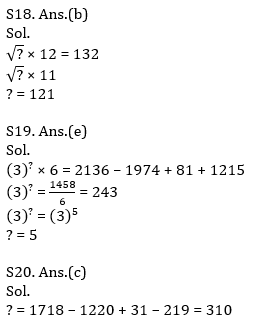Latest Banking jobs   »   Quantitative Aptitude Quiz For IBPS RRB...

# Quantitative Aptitude Quiz For IBPS RRB PO, Clerk Prelims 2021- 6th June

Directions (1-5): In each of these questions, two equations (I) and (II) are given. You have to solve both the equations and give answer
(a) if x>y
(b) if x≥y
(c) if x<y
(d) if x ≤y
(e) if x = y or no relation can be established between x and y.

Q1. I. 6x² + 11x – 35 = 0
II. 2y² + 15y + 28 = 0

Q2. I. x² – 17x = 0
II. y³ – 4913 = 0

Q3. I. (x – 5)² – 100 = 0
II. y² + 16y = 5(y – 6)

Q4. I. 11x² + 18x + 7 = 0
II. 22y² + 25y + 7 = 0

Q5. I. 8x + 7y = 38
II. 3x – 5y = –1

Directions (6 – 10): What will come in the place of question (?) mark in the following number series:

Q6. 180, 185, 193, 207, 233, ?
(a) 268
(b) 278
(c) 273
(d) 295
(e) 283

Q7. 160, 82, 84, 128, ?, 647
(a) 342
(b) 314
(c) 292
(d) 258
(e) 284

Q8. ?, 334, 166, 82, 40, 19
(a) 570
(b) 558
(c) 670
(d) 640
(e) 645

Q9. 1250, 961, 736, 567, 446, ?
(a) 365
(b) 385
(c) 280
(d) 340
(e) 345

Q10. 120, 120, 126, 146, 188, ?
(a) 240
(b) 220
(c) 225
(d) 275
(e) 260

Directions (11-15): Find the approximate value of question marks (?) in following questions?

Q11. 27.82% of 449.92 + ?% of 1199.86 = 199.89 + 225.92
(a) 45
(b) 25
(c) 40
(d) 28
(e) 35

Q12. 1729.79 + 1269.89 + ? = 249.91 × 19.83
(a) 3000
(b) 2800
(c) 1800
(d) 2000
(e) 2200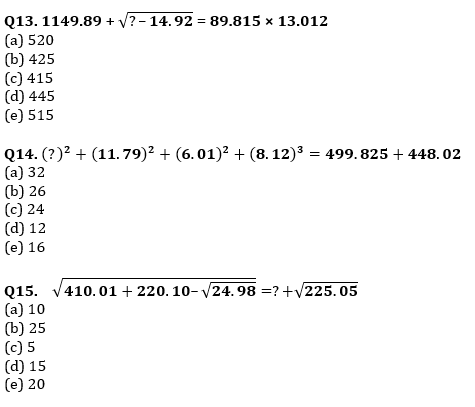Directions (16-20): Find the exact value of the following questions: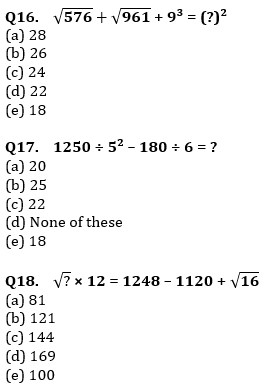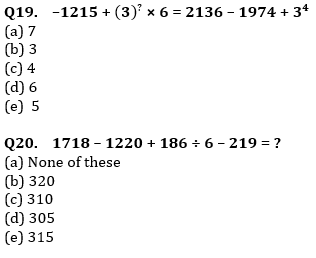Solutions